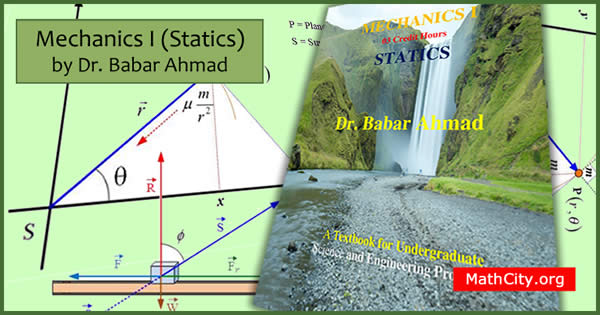# Mechanics I (Statics) by Dr Babar AhmadWe are very thankful to Dr Babar Ahmad for sharing his book on MathCity.org. This book is very helpful for undergraduate students of Science and Engineering Programs.

This book is shared by the permission of the author and he keeps the copyright of the book.

 Name Mechanics I (Statics) Dr. Babar Ahmad 398 pages PDF (see Software section for PDF Reader) 3.83 MB

Mechanics is one of the most important course in maximum disciplines of science and engineering. No matter what your interest in science or engineering, mechanics will be important for you. Mechanics is a branch of physics which deals with the bodies at rest and in motion. During the early modern period, scientists such as Galileo, Kepler, and Newton laid the foundation for what is now known as classical mechanics. Hence there is an extensive use of mathematics in its foundation.

Mechanics is core course for undergraduate Mathematics, Physics and many engineering disciplines. It appears under different names as Analytical/Classical Mechanics, Theoretical Mechanics, Mechanics I, Mechanics II, Mechanics III, Analytical Dynamics.

This textbook is designed to support teaching activities in Theoretical Mechanics specially Statics. It covers the contents of “Mechanics” for many undergraduate science and engineering programs. It presents simply and clearly the main theoretical aspects of mechanics. It is assumed that the students have completed their courses in Calculus, Linear Algebra and Differential Equations. This book also lay the foundations for further studies in physics, physical sciences, and engineering.

• Units and Dimensions of Physical Quantities
• International System of Units (SI)
• Units outside the SI
• Dimensions
• Scalars and Vectors
• Scalar Quantities or Scalars
• Vector Quantities or Vectors
• Geometric Representation of a Vector
• Free-body diagram
• System of Vectors
• Scalar and Vector Products of Two Vectors
• Scalar or Dot Product of Three Vectors
• Addition of Vectors
• Resultant of Coplanar Vectors
• Resultant of Concurrent and Coplanar Vectors
• Resultant of Three Concurrent and Non-coplanar Vectors
• Vector Field
• Composition and Resolution of Forces
• Equilibrium
• Friction
• Linear Momentum, Impulse and Collision
• Angular Momentum
• Work Energy and Conservative Force
• Virtual Displacement and Virtual Work
• Centers of Mass and Gravity
• Moments and Products of Inertia